RD Sharma Class 12 Solutions Chapter 33 Binomial Distribution Ex 33.2 part of RD Sharma Class 12 Solutions. Here we have given RD Sharma Class 12 Solutions Chapter 33 Binomial Distribution Ex 33.2.

Here you can get free RD Sharma Solutions for Class 12 Maths of Chapter 33 Binomial Distribution Exercise 33.2. All RD Sharma Book Solutions are given here exercise wise for Binomial Distribution. RD Sharma Solutions are helpful in the preparation of several school level, graduate and undergraduate level competitive exams. Practicing questions from RD Sharma Mathematics Solutions for Class 12 Chapter 33 Binomial Distribution is proven to enhance your math skills.

 Class: 12th Class Chapter: Chapter 33 Name: Binomial Distribution Exercise: Exercise 33.2

## RD Sharma Class 12 Solutions Chapter 33 Binomial Distribution Ex 33.2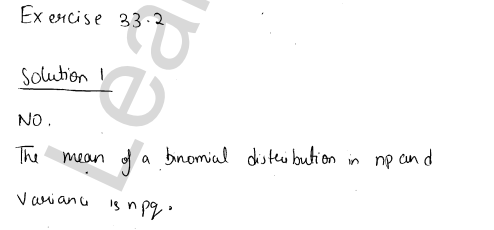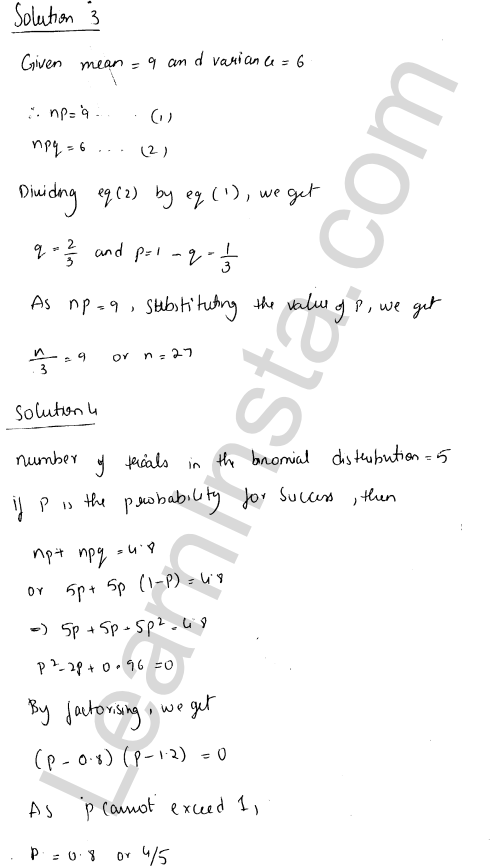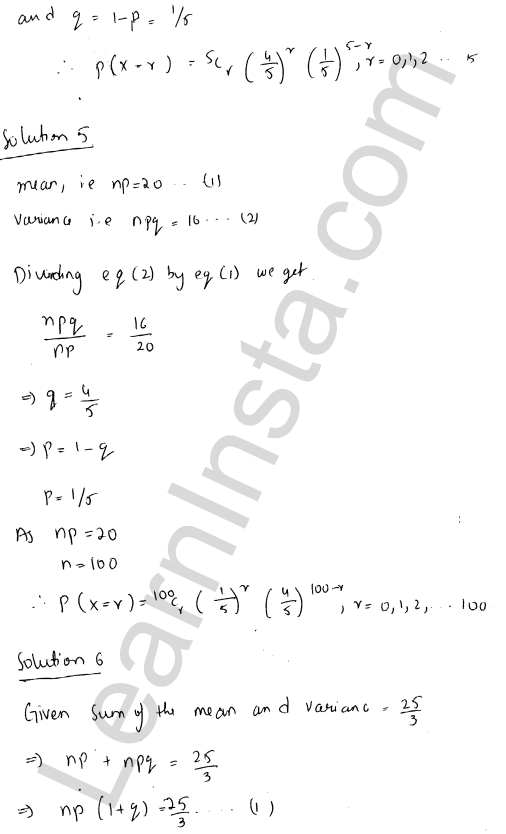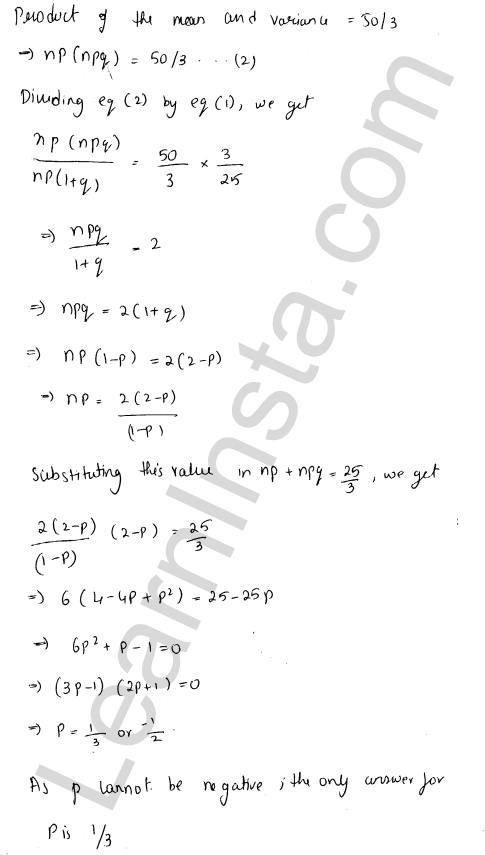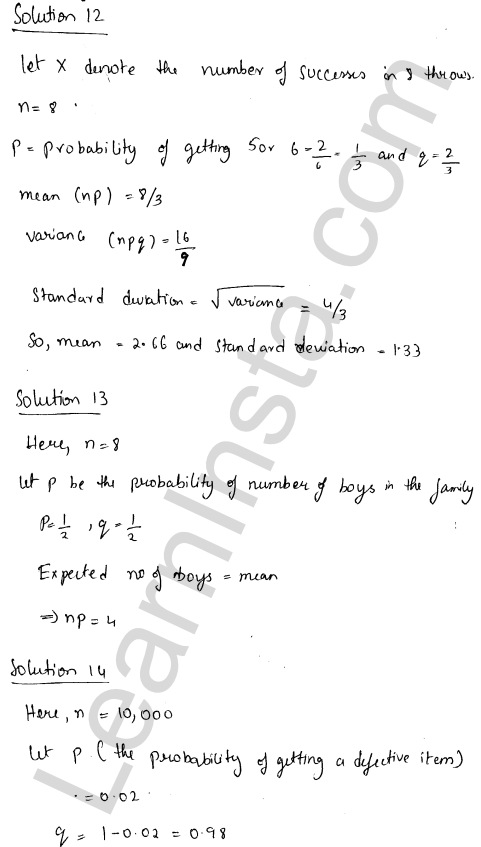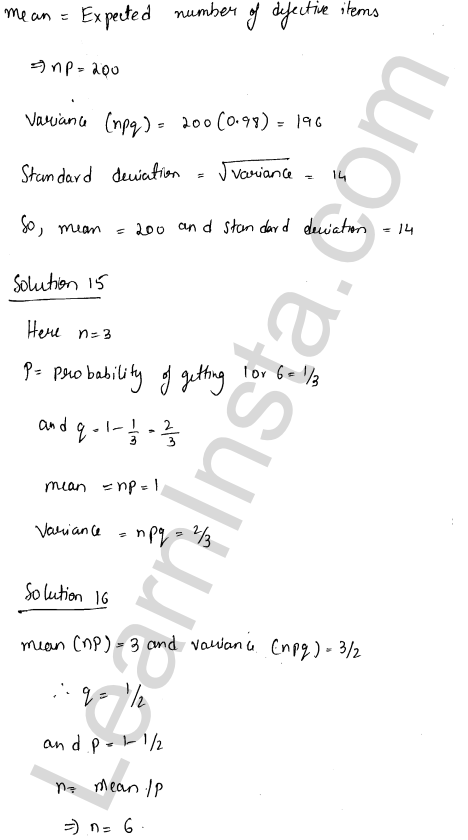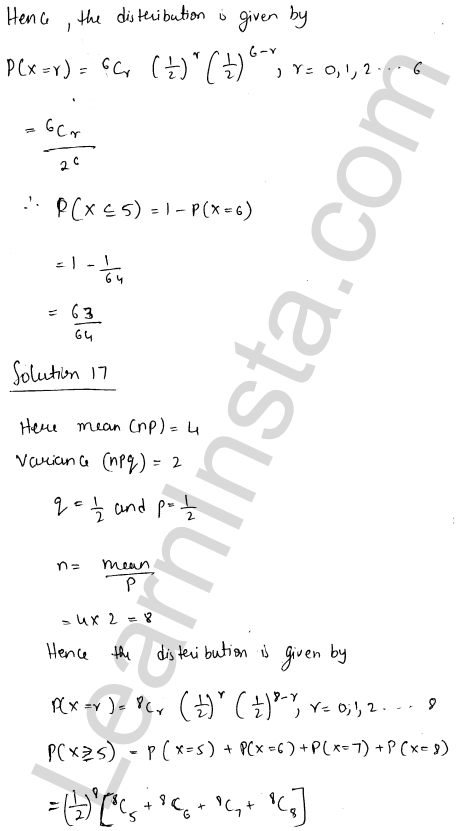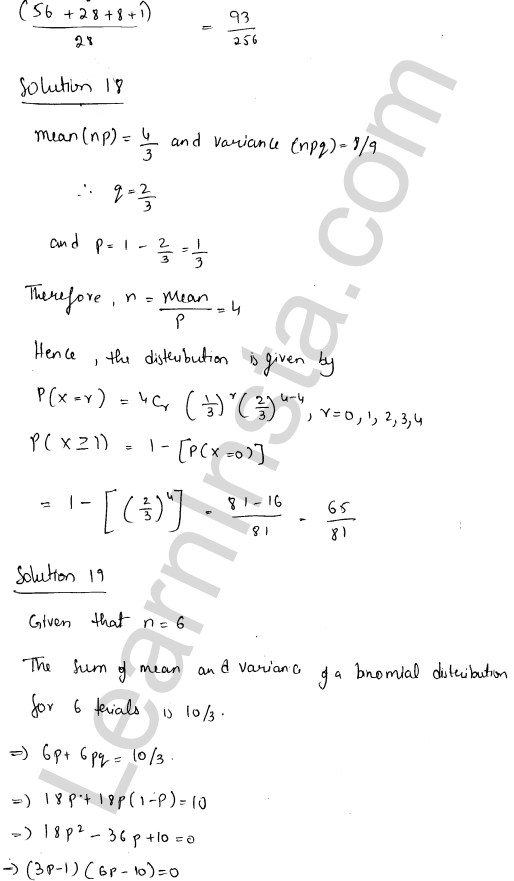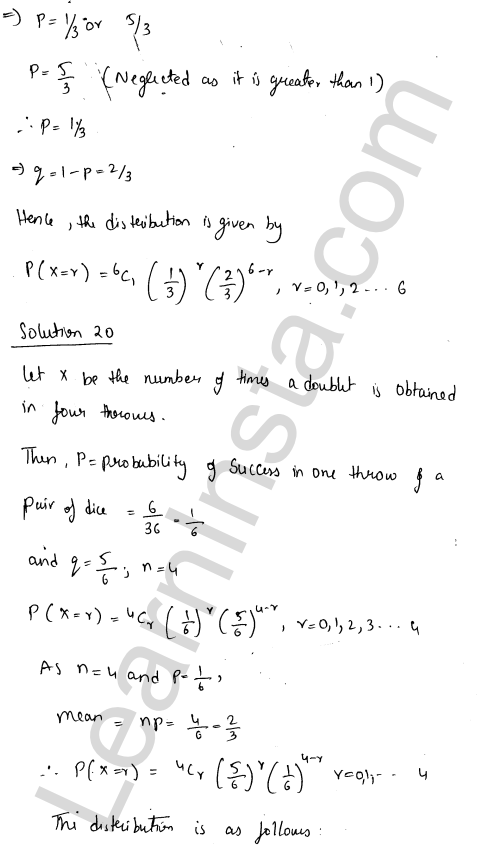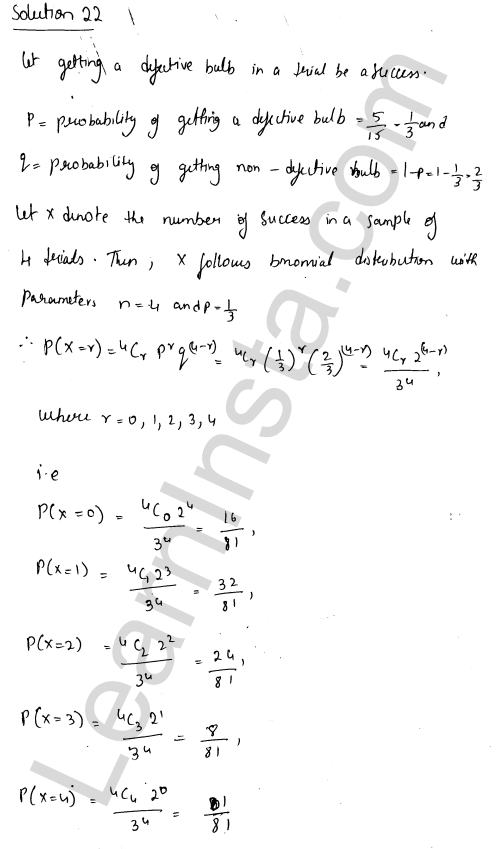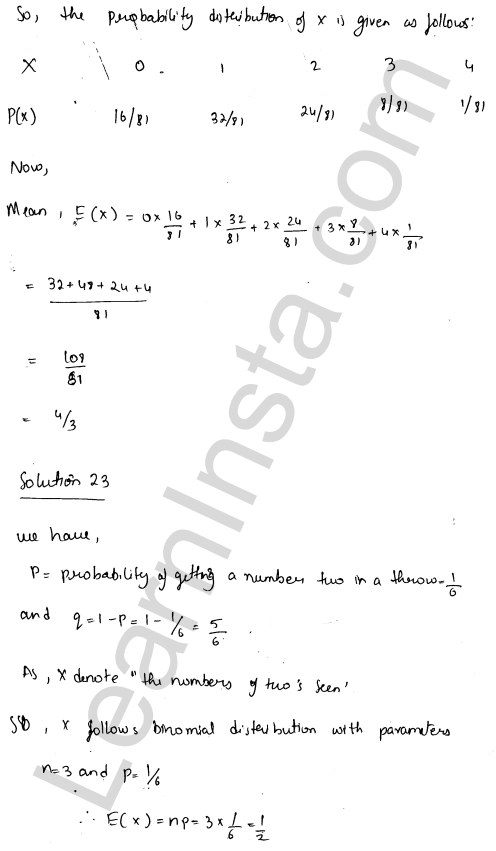We hope the RD Sharma Class 12 Solutions Chapter 33 Binomial Distribution Ex 33.2, help you. If you have any query regarding RD Sharma Class 12 Solutions Chapter 33 Binomial Distribution Ex 33.2, drop a comment below and we will get back to you at the earliest.